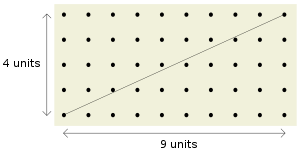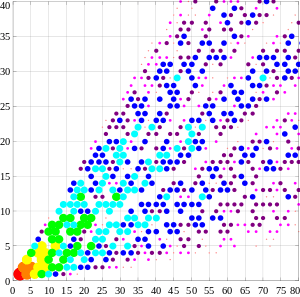# Coprime integersLook up coprime in Wiktionary, the free dictionary.

In number theory, two integers a and b are said to be relatively prime, mutually prime, or coprime (also spelled co-prime) if the only positive integer that divides both of them is 1. That is, the only common positive factor of the two numbers is 1. This is equivalent to their greatest common divisor being 1. The numerator and denominator of a reduced fraction are coprime. In addition toandthe notationis sometimes used to indicate that a and b are relatively prime.

For example, 14 and 15 are coprime, being commonly divisible by only 1, but 14 and 21 are not, because they are both divisible by 7. The numbers 1 and −1 are the only integers coprime to every integer, and they are the only integers to be coprime with 0.

A fast way to determine whether two numbers are coprime is given by the Euclidean algorithm.

The number of integers coprime to a positive integer n, between 1 and n, is given by Euler's totient function (or Euler's phi function) φ(n).

A set of integers can also be called coprime if its elements share no common positive factor except 1. A set of integers is said to be pairwise coprime if a and b are coprime for every pair (a, b) of different integers in it.

## Properties

A number of conditions are individually equivalent to a and b being coprime:

As a consequence of the third point, if a and b are coprime and br bs (mod a), then r s (mod a). That is, we may "divide by b" when working modulo a. Furthermore, if b1 and b2 are both coprime with a, then so is their product b1b2 (modulo a it is a product of invertible elements, and therefore invertible); this also follows from the first point by Euclid's lemma, which states that if a prime number p divides a product bc, then p divides at least one of the factors b, c.

As a consequence of the first point, if a and b are coprime, then so are any powers ak and bl.

If a and b are coprime and a divides the product bc, then a divides c. This can be viewed as a generalization of Euclid's lemma.Figure 1. The numbers 4 and 9 are coprime. Therefore, the diagonal of a 4 x 9 lattice does not intersect any other lattice points

The two integers a and b are coprime if and only if the point with coordinates (a, b) in a Cartesian coordinate system is "visible" from the origin (0,0), in the sense that there is no point with integer coordinates on the line segment between the origin and (a, b). (See figure 1.)

In a sense that can be made precise, the probability that two randomly chosen integers are coprime is 6/π2 (see pi), which is about 61%. See below.

Two natural numbers a and b are coprime if and only if the numbers 2a 1 and 2b 1 are coprime. As a generalization of this, following easily from the Euclidean algorithm in base n > 1:## Coprimality in sets

A set of integers S = {a1, a2, .... an} can also be called coprime or setwise coprime if the greatest common divisor of all the elements of the set is 1. For example, the integers 6, 10, 15 are coprime because 1 is the only positive integer that divides all of them.

If every pair in a set of integers is coprime, then the set is said to be pairwise coprime (or pairwise relatively prime, mutually coprime or mutually relatively prime). Pairwise coprimality is a stronger condition than setwise coprimality; every pairwise coprime finite set is also setwise coprime, but the reverse is not true. For example, the integers 4, 5, 6 are (setwise) coprime (because the only positive integer dividing all of them is 1), but they are not pairwise coprime (because gcd(4, 6) = 2).

The concept of pairwise coprimality is important as a hypothesis in many results in number theory, such as the Chinese remainder theorem.

It is possible for an infinite set of integers to be pairwise coprime. Notable examples include the set of all prime numbers, the set of elements in Sylvester's sequence, and the set of all Fermat numbers.

## Coprimality in ring ideals

Two ideals A and B in the commutative ring R are called coprime (or comaximal) if A + B = R. This generalizes Bézout's identity: with this definition, two principal ideals (a) and (b) in the ring of integers Z are coprime if and only if a and b are coprime. If the ideals A and B of R are coprime, then AB = AB; furthermore, if C is a third ideal such that A contains BC, then A contains C. The Chinese remainder theorem can be generalized to any commutative ring, using coprime ideals.

## Probabilities

Given two randomly chosen integers a and b, it is reasonable to ask how likely it is that a and b are coprime. In this determination, it is convenient to use the characterization that a and b are coprime if and only if no prime number divides both of them (see Fundamental theorem of arithmetic).

Informally, the probability that any number is divisible by a prime (or in fact any integer)is; for example, every 7th integer is divisible by 7. Hence the probability that two numbers are both divisible by p is, and the probability that at least one of them is not is. Any finite collection of divisibility events associated to distinct primes is mutually independent. For example, in the case of two events, a number is divisible by primes p and q if and only if it is divisible by pq; the latter event has probability 1/pq. If one makes the heuristic assumption that such reasoning can be extended to infinitely many divisibility events, one is led to guess that the probability that two numbers are coprime is given by a product over all primes,Here ζ refers to the Riemann zeta function, the identity relating the product over primes to ζ(2) is an example of an Euler product, and the evaluation of ζ(2) as π2/6 is the Basel problem, solved by Leonhard Euler in 1735.

There is no way to choose a positive integer at random so that each positive integer occurs with equal probability, but statements about "randomly chosen integers" such as the ones above can be formalized by using the notion of natural density. For each positive integer N, let PN be the probability that two randomly chosen numbers inare coprime. Although PN will never equalexactly, with work one can show that in the limit as, the probabilityapproaches.

More generally, the probability of k randomly chosen integers being coprime is 1/ζ(k).

## Generating all coprime pairsThe order of generation of coprime pairs by this algorithm. First node (2,1) is marked red, its three children are shown in orange, third generation is yellow, and so on in the rainbow order.

All pairs of positive coprime numbers(with) can be arranged in two disjoint complete ternary trees, one tree starting from(for even-odd and odd-even pairs), and the other tree starting from(for odd-odd pairs). The children of each vertexare generated as follows:

Branch 1:Branch 2:Branch 3:This scheme is exhaustive and non-redundant with no invalid members.

## References

1. Eaton, James S. Treatise on Arithmetic. 1872. May be downloaded from: https://archive.org/details/atreatiseonarit05eatogoog
2. G.H. Hardy; E. M. Wright (2008). An Introduction to the Theory of Numbers (6th ed.). Oxford University Press. p. 6. ISBN 978-0-19-921986-5.
3. Graham, R. L.; Knuth, D. E.; Patashnik, O. (1989), Concrete Mathematics, Addison-Wesley
4. This theorem was proved by Ernesto Cesàro in 1881. For a proof, see G.H. Hardy; E. M. Wright (2008). An Introduction to the Theory of Numbers (6th ed.). Oxford University Press. ISBN 978-0-19-921986-5., theorem 332.
5. Saunders, Robert & Randall, Trevor (July 1994), "The family tree of the Pythagorean triplets revisited", Mathematical Gazette, 78: 190–193, doi:10.2307/3618576.
6. Mitchell, Douglas W. (July 2001), "An alternative characterisation of all primitive Pythagorean triples", Mathematical Gazette, 85: 273–275, doi:10.2307/3622017.